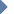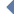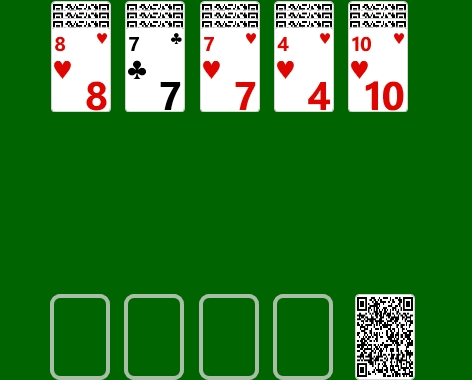SoftwareHomeQ&ASupportPatternA deck of Kabufuda cards (40 cards, with designs representing the numbers 1 through 10, there are four cards for each number) divided equally. The first part of deck is 5 playing piles. The second part is stock.Tap on the stock to deal the 4 cards. This is "model". Object of the game is discard all the cards by using combinations of the model cards.Calculate the sum of the model cards. If the sum of the values of the cards is 10 or upper then used only lowest order digit. For example: 7+5+5+9 = 26, so value = 6; 3+3+1+2 = 9 so value = 9. Try to choose from two and up to five cards on the playing piles. Their sum is calculated by the same rules as for the model cards. If the sum are equal to the sum of model cards these cards can be removed by pressing the "OK" button. If one's playing pile is empty, this place is allowed to move the card from the other pile. Continue in this manner until the stock is exhausted or while it is possible to remove the cards.This image is a schematic representation, not a screenshot from the game. Screenshots are platform specific. You can see screenshots in the store.